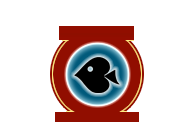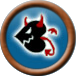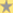.form_login_top { font-family: Verdana; font-size: 7pt; color: #eee; border: 1px solid #eee; height: 17px; width: 80px; background-color: #7a3c2c; padding: 2px; } .form_login_top2 { font-family: Verdana; font-size: 7pt; color: #eee; border: 1px solid #eee; height: 17px; width: 80px; background-color: #7a3c2c; padding: 2px; }Home  |  Poker Blogs  |  Poker Forum  |  Poker Articles  |  Rakeback  |  Login  |  RegisterPoker NewsLP Pokerstars Avatars:a.textLinkTop{color: #; font-size:12px; font-weight:bold; text-decoration: none; } a.textLinkTop:hover {color:#;}# supNew to LiquidPoker? Register here for free!
random HU NL thoughts
fira, Jan 08 2016

200 deep HU

IP 3x
OOP 3bet to 12x
IP 4bet to 36x

Can OOP call here with a hand like 65s? Intuitively I think the answer is yes, but it could also be no.

If the answer is no, how would we balance our range on boards that favor 65? (66x, 55x, any kind of board where 6 and/or 5 is a straight). Is it not something to worry about at all, since these kinds of boards are rare enough and the SPR is low enough for 65 to not matter?

If at 200 deep we can't call, there is surely a stack depth at which we can (and must?) call, despite being OOP and facing a rather large 4bet. However I often feel like a fish for calling big 4bets OOP with a drawing hand. I guess it really depends exactly how deep players are?

(65s is mostly just an example hand, it could be anything from 22 to AK, although with 22 or AK I feel a little better making the call than with 65s.)0 votes

Comments (5)

Poker Calculations: Video Poker
fira, Dec 06 2015

((Just some random poker calculations I did via brute force. I'd appreciate it if someone more knowledgeable could confirm the legitimacy of these calculations, whether it be the results or the "methods"!))

Game: Video Poker Jacks or Better

Payout chart:
~Royal flush 250x
~Straight flush 50x
~4OAK 25x
~Full House 9x
~Flush 6x
~Straight 4x
~3OAK 3x
~2Pair 2x
~JJ+ 1x

Our Hand: Ad Jc Xx Yy Zz

What do we do? Options:
~hold Ad
~hold Ad, Jc

========================================================================================================================
Option 1: hold Ad:
if we hold just Ad, we discard the other four cards and draw 4 new cards
our total EV should equal:
(chance of getting royal flush * 250) +
(chance of getting straight flush * 50) +
(chance of getting 4OAK * 25) +
(chance of getting full house * 9) +
(chance of getting flush * 6) +
(chance of getting straight * 4) +
(chance of getting 3OAK * 3) +
(chance of getting 2Pair * 2) +
(chance of getting JJ+ * 1)

chance of getting royal flush = 4/50 * 3/49 * 2/48 * 1/47 = 0.00000434216 = 1/230,300
chance of getting straight flush = 4/50 * 3/49 * 2/48 * 1/47 = 0.00000434216 = 1/230,300

chance of getting 4OAK =
--4 ways to get four Aces--
{A, A, A, X}
{A, A, X, A}
{A, X, A, A}
{X, A, A, A} (3/50 * 2/49 * 1/48) = 5.10204082E-5 = 1/19,600
--1 way to get four Xs--
{X, X, X, X} (47/50 * 3/49 * 2/48 * 1/47) = 5.10204082E-5 = 1/19,600
Total = 5 * 5.10204082E-5 = 0.000255102041 = 1/3,920

chance of getting full house =
--6 ways to get Aces full--
{A, A, X, X}
{A, X, A, X}
{A, X, X, A}
{X, A, A, X}
{X, A, X, A}
{X, X, A, A} (47/50 * 3/49 * 3/48 * 2/47) = 0.00015306122 = 1/6,533
--4 ways to get X's full--
{A, X, X, X}
{X, A, X, X}
{X, X, A, X}
{X, X, X, A} (47/50 * 3/49 * 2/48 * 3/47) = 0.00015306122 = 1/6,533
Total = 10 * 0.00015306122 = 0.0015306122 = 1/653

chance of getting flush =
{Wd, Xd, Yd, Zd} (12/50 * 11/49 * 10/48 * 9/47) = 0.00214937038 = 1/465

chance of getting straight =
--2 ways to get straight--
{2,3,4,5} (16/50 * 12/49 * 8/48 * 4/47) = 0.00111159357 = 1/899
{KQT,KQT,KQT,J} (12/50 * 8/49 * 4/48 * 3/47) = 0.00020842379 = 1/4798
{KQT,KQT,J,KQT}
{KQT,J,KQT,KQT}
{J,KQT,KQT,KQT}
Total = 0.00111159357 + (4 * 0.00020842379) = 0.00194528873 = 1/514

chance of getting 3OAK =
--6 ways to get trip Aces--
{A, A, X, Y}
{A, X, A, Y}
{A, X, Y, A}
{X, A, A, Y}
{X, A, Y, A}
{X, Y, A, A} (47/50 * 43/49 * 3/48 * 2/47) = 0.00219387755 = 1/456
--4 ways to get trip Xs--
{X, X, X, Y}
{X, X, Y, X}
{X, Y, X, X}
{Y, X, X, X} (47/50 * 43/49 * 3/48 * 2/47) = 0.00219387755 = 1/456
Total = 10 * 0.00219387755 = 0.0219387755 = 1/46

chance of getting 2Pair =
--10 ways to get Aces up--
{A, X, X, Y}
{A, X, Y, X}
{A, Y, X, X}
{X, A, X, Y}
{Y, A, X, X}
{X, X, A, Y}
{X, Y, A, X}
{X, X, Y, A}
{X, Y, X, A}
{Y, X, X, A} (47/50 * 43/49 * 3/48 * 3/47) = 0.00329081632 = 1/304
--6 ways to get other 2Pair--
{X, X, Y, Y}
{X, Y, X, Y}
{X, Y, Y, X}
{Y, X, X, Y}
{Y, X, Y, X}
{Y, Y, X, X} (47/50 * 3/49 * 43/48 * 3/47) = 0.00329081632 = 1/304
Total = 16 * 0.00329081632 = 0.05265306112 = 1/19

chance of getting JJ+ =
--4 ways to get pair of Aces--
{A, X, Y, Z}
{X, A, Y, Z}
{X, Y, A, Z}
{X, Y, Z, A} = (47/50 * 43/49 * 39/48 * 3/47) = 0.04278061224 = 1/23
--6 ways to get pair of Kings--
{K, K, X, Y}
{K, X, K, Y}
{K, X, Y, K}
{X, K, K, Y}
{X, K, Y, K}
{X, Y, K, K} = (43/50 * 40/49 * 4/48 * 3/47) = 0.00373425966 = 1/268
--6 ways to get pair of Queens--
same as Kings
--6 ways to get pair of Jacks--
{X, Y, J, J} = (43/50 * 40/49 * 3/48 * 2/47) = 0.00186712983 = 1/536
Total = (0.04278061224 * 4) + (0.00373425966 * 12) + (0.00186712983 * 6) = 0.22713634386 = 1/4.4

Total EV of keeping just Ad =
(0.00000434216 * 250) +
(0.00000434216 * 50) +
(0.00025835866 * 25) +
(0.0015306122 * 9) +
(0.00214937038 * 6) +
(0.00194528873 * 4) +
(0.0219387755 * 3) +
(0.05265306112 * 2) +
(0.22713634386 * 1) = 0.4404732941
========================================================================================================================
========================================================================================================================
Option 2 - hold Ad, Jc:
if we hold both Ad and Jc, we discard the other three cards and draw 3 new cards
our total EV should equal:
(chance of getting royal flush * 250) +
(chance of getting straight flush * 50) +
(chance of getting 4OAK * 25) +
(chance of getting full house * 9) +
(chance of getting flush * 6) +
(chance of getting straight * 4) +
(chance of getting 3OAK * 3) +
(chance of getting 2Pair * 2) +
(chance of getting JJ+ * 1)

chance of getting royal flush = 0
chance of getting straight flush = 0
chance of getting 4OAK = (3/50 * 2/49 * 1/48) * 2 = 0.00010204081 = 1/9800

chance of getting full house =
--3 ways to get Aces full of Jacks--
{A, A, J}
{A, J, A}
{J, A, A}
--3 ways to get Jacks full of Aces--
{A, J, J}
{J, A, J}
{J, J, A} = (3/50 * 2/49 * 3/48) = 0.00015306122 = 1/6,533
Total = 6 * 0.00015306122 = 0.00091836732 = 1/1089

chance of getting flush = 0

chance of getting straight =
{KQT, KQT, KQT} (12/50 * 8/49 * 4/48) = 0.00326530612 = 1/306

chance of getting 3OAK =
--3 ways to get trip Aces--
{A, A, X}
{A, X, A}
{X, A, A}
--3 ways to get trip Jacks--
{J, J, X}
{J, X, J}
{X, J, J} = (44/50 * 3/49 * 2/48) = 0.00224489795 = 1/445
--1 way to get trip Xs--
{X, X, X} = (44/50 * 3/49 * 2/48)
Total = 7 * 0.00224489795 = 0.0157142857 = 1/64

chance of getting 2Pair =
--3 ways to get Aces up (non-Jacks)--
{A, X, X}
{X, A, X}
{X, X, A} = (44/50 * 3/49 * 3/48) = 0.00336734693 = 1/297
--3 ways to get Jacks up (non-Aces)--
{J, X, X}
{X, J, X}
{X, X, J}
--6 ways to get Aces and Jacks--
{A, J, X}
{A, X, J}
{J, A, X}
{J, X, A}
{X, A, J}
{X, J, A} = (44/50 * 3/49 * 3/48) = 0.00336734693 = 1/297
Total = 12 * 0.00336734693 = 0.04040816316 = 1/25

chance of getting JJ+ =
--3 ways to get pair of Aces--
{A, X, Y}
{X, A, Y}
{X, Y, A} = (44/50 * 40/49 * 3/48) = 0.04489795918 = 1/22
--3 ways to get pair of Jacks--
{J, X, Y}
{X, J, Y}
{X, Y, J}
--3 ways to get pair of Kings--
{K, K, X}
{K, X, K}
{X, K, K} = (40/50 * 4/49 * 3/48) = 0.00408163265 = 1/245
--3 ways to get pair of Queens--
{Q, Q, X}
{Q, X, Q}
{X, Q, Q}
Total = (0.04489795918 * 6) + (0.00408163265 * 6) = 0.29387755098 = 1/3.4

Total EV of keeping Ad and Jc =
(0 * 250) +
(0 * 50) +
(0.00010204081 * 25) +
(0.00091836732 * 9) +
(0 * 6) +
(0.00326530612 * 4) +
(0.0157142857 * 3) +
(0.04040816316 * 2) +
(0.29387755098 * 1) = 0.44571428501
========================================================================================================================

Comparison--------------Hold Ad-------------Hold Ad, Jc--------
Royal flush EV----------0.00108554----------0------------------
Straight flush EV-------0.000217108---------0------------------
4OAK EV-----------------0.0064589665--------0.00255102025------
Full house EV-----------0.0137755098--------0.00826530588------
Flush EV----------------0.01289622228-------0------------------
Straight EV-------------0.00778115492-------0.01306122448------
3OAK EV-----------------0.0658163265--------0.0471428571-------
2Pair EV----------------0.10530612224-------0.08081632632------
JJ+ EV------------------0.22713634386-------0.29387755098------
Total EV----------------0.4404732941--------0.44571428486------0 votes

Comments (12)

Down so much from tournaments
fira, Nov 19 2015

I've been playing some PLO8 tournaments on Bovada, and my ROI is currently at about -75%...

At this point it would take more than a first place prize just to get back to even. Depressing ~_~0 votes

Comments (12)

Next PageCopyright © 2019. LiquidPoker.net All Rights Reserved Contact Advertise Sitemap